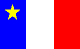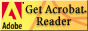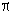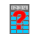# Preparing for University Calculus

Are you going to enrol in an Atlantic region university this year? Are you planning to take science courses? Then there is a good chance that you will have to pass a course in calculus as a requirement for your degree or a prerequisite for other courses. This page is here to help you prepare for that calculus course, enjoy it, and do well in it.
 Contents What math will I need in university? What is calculus? Why is calculus important? What background will I need? What is taking university calculus like? HELP! I have to write a placement test! HELP! They want to take away my calculator! Get the booklet "Preparing for University Calculus" Note: This is available online in three forms. For each one you will need the associated viewer! These can be downloaded free of charge. Find out more about the booklet here Get the booklet in PostScript format. Get the booklet in PDF format Lire cette pageen français Read the PS version with GhostView . You will need to run Ghostview once after installation, to configure it, before viewing booklet! To read PDF files,## What math will I need in university?

The answer to this depends on what you are taking, and you should check with the university or universities that you are thinking of attending. As a general rule, however, if you are planning to get a degree in science, you will probably need one or more courses in calculus (some universities permit programming or statistics instead in some cases). If you are taking courses in the social sciences or life sciences, you will probably need one or more courses in statistics; and degrees in physics, engineering, astronomy, mathematics, or computing science (and some other subjects) will require additional mathematics courses beyond the first year level.

Some departments may teach their own courses in calculus, linear algebra, or statistics. In this case, you may not need to take the standard first-year courses offered by the mathematics department.

## What is calculus?

Calculus is a branch of mathematics that deals with rates of change. Its roots go back as far as Ancient Greece and China, but calculus as we know it today began with Newton and Leibnitz in the 17th century. Today it is used extensively in many areas of science.

Basic ideas of calculus include the idea of limit , derivative , and integral . The derivative of a function is its instantaneous rate of change, with respect to something else. Thus, the derivative of height , (with respect to position) is slope ; the derivative of position , (with respect to time) is velocity ; and the derivative of velocity (with respect to time) is acceleration.

The integral of a function can be thought of as the area under its graph, or as a sort of total over time. Thus, the integral of slope is (up to a constant) height ; the integral of velocity is, up to a constant, position; and the integral of acceleration (with respect to time) is velocity. As you may have guessed, integrals and derivatives are related, and are in a sense opposites.

Now, many functions (though not all) can be represented by algebraic expressions. For instance, the area of a circle is related to its radius by the formula A=r2; and the distance that a body falls in a time t, starting at rest, is given by x = 1/2 a t2. Given such an expression, calculus allows us to find expressions for the integral and derivative of the function, when they exist.

## Why is calculus important?

In the sciences, many processes involving change, or related variables, are studied. If these variables are linked in a way that involves chance, and significant random variation, statistics is one of the main tools used to study the connections. But, in cases where a deterministic model is at least a good approximation, calculus is a powerful tool to study the ways in which the variables interact. Situations involving rates of change over time, or rates of change from place to place, are particularly important examples.

Physics, astronomy, mathematics, and engineering make particularly heavy use of calculus; it is difficult to see how any of those disciplines could exist in anything like its modern form without calculus. However, biology, chemistry, economics, computing science, and other sciences use calculus too. Many faculties of science therefore require a calculus course from all their students; in other cases you may be able to choose between, say, calculus, statistics, and computer programming.

It should be understood that there is more to mathematics than calculus. Some mathematics courses may have first-year calculus as a prerequisite to ensure that students taking those courses have a certain level of mathematical sophistication, but may not actually use calculus at all.

## What background do I need?

You should have taken Grade 12 precalculus mathematics, or an equivalent course, and understood the material. (UPEI and MUN currently require slightly less, but the precalculus course is advisable in any case.) The second part of this booklet gives some examples of the sort of thing that you should be able to do.

Note that some choices of math course at the Grade 10 or Grade 11 level may make it difficult or impossible for you to take precalculus math. Do not throw away your options by a careless choice of courses!

• In New Brunswick, you may take Math 111 (preferred) or Math 112 in Grade 11, but you must take both semesters. You may take Math 121 (preferred) or Math 122 in Grade 12, and in either case you must also take Math 120.

• In Newfoundland and Labrador, you will need to take Math 1204, 2205, 3205, and 3207.

• In Nova Scotia, you will need to take Math 10 rather than Math Foundations 10 in Grade 10, and then advanced Math 11 and Math 12 , (not "academic") ending up with Precalculus Math 12 .

• In Prince Edward Island, you will need to take both Math 521A and Math 521B in Grade 11. In Grade 12 you will need Math 621B ( not Math 621A), and MAT611B .
You do not need a high school calculus course; if you have taken one, you should not assume that you can skip classes, or not study, during the first part of the course. University calculus goes deeper than most courses in high schools do, even if they appear to cover the same material. If you have been lucky enough to have a calculus course in high school, consider it as a preview of the upcoming feature that you are now about to see! And remember to review your algebra, as you may have had extra time to forget it.

## What is taking university calculus like?

You will probably find that university calculus is faster-paced than your high-school courses. At most universities the lecture sections will be bigger -- possibly over a hundred students -- and the professor will not be able to slow the class to the pace of the slowest students. You -- and you alone -- will be responsible for handing work in on time, and for being present for classes, tests, and exams.

This is not a course that can be passed by just memorizing everything; you have to understand it. This won't happen instantly, and the instructor cannot make it happen; you have to do that yourself, and be an active participant in the course. If you make this effort consistently through the term, you will find that it pays off.

The course material consists of a rather small number of big ideas, and a moderate number of formulae you will need to know, not hundreds of short cuts and special rules. A common mistake, especially with word problems, is trying to learn one ``plug-in'' rule for each different kind of problem that you might encounter. Don't do that; instead, try to understand the underlying patterns.

Here are the stages by which you will learn and master a new idea in calculus.

1. First, you should read ahead in the textbook, so that you have at least a rough idea of what the instructor is going to say. This will help you follow the lecture and make note-taking easier.
2. In the lecture, the instructor will introduce the new idea, give some examples, and perhaps explain how it fits in with other ideas or give some problem-solving tips.
3. At this point, a few students think the process is over till the final exam and that if they don't know everything then, it will be the instructor's fault. Not so; the ball is back in your court! Work on problems , do the assignments (including additional study problems if you need to, whether assigned or not), and make sure that you understand what you are doing. It's better to work for an hour or so several times a week than in one killer session. This is the heart of the learning process; the lecture is just to help you get started.
4. If you don't understand something, decide what it is that you don't understand and go for help. Go prepared to tell the instructor (or friend, tutor, learning center person, etc.) what it is that you don't understand. (``Everything'' is not helpful.) On the other hand, do not go along demanding that the instructor tell you how to answer Question 6.11 and refusing to listen to any explanation of why it's done that way.
5. Finally, your knowledge will be tested on quizzes, midterms, or final examinations. The exams will probably make up the majority of your grade. If you have handed in copied, half-understood assignments, you will lose more marks at exam time than you gained in the short term. But if you kept up with the lectures and assignments, making sure you understood each bit before going on, you will probably do well.
If you find yourself falling behind, you will have to catch up. Don't panic - catching up is not impossible. There are various resources to help.Yourself. If you are falling behind and not putting plenty of time in studying - say five or six hours outside class per week for each course - the solution may be as near as your desk and textbook.Your textbook contains hundreds of worked examples, and thousands of problems. Usually, about half of them have answers in the back of the book; and you may be able to buy a study guide that shows the working of those problems in more detail. You can also get other books such as ``Schaum's Outline of Calculus'' containing more worked problems.Your instructor will have office hours during which you can go for help. Try to figure out ahead of time what you need help with; you will get more out of the visit.Discuss your problem with your classmates. This is not the same thing as copying their assignments, of course! University penalties for cheating are severe, and can include expulsion.You might want to hire a tutor . Do not try to get the tutor to do your assignments for you; you will not be able to bring the tutor into the exam with you! Get the tutor to make sure you understand the material.Your university may have a math learning center , group tutoring sessions, or other resources that you can use. Take advantage of them!Your university or student union may well have workshops on effective studying, effective note-taking, exam nerves, etc. There will also be counselling available for other problems that might interfere with your study.
Back to the top

## HELP! I have to write a placement test!

Many Atlantic-area universities have found that incoming students are not uniformly well-prepared for calculus. Many students are very well prepared, and ready to start the usual calculus curriculum; but some are not. As a result, placement tests are common, and at some universities, if you do not pass the placement test, you will be required to take a remedial course covering important material from the high school curriculum before you can take calculus. At other universities you may just be advised to take the remedial course, or allowed into a slow-moving section that takes two semesters to cover the first semester's material.

This is to stop students from starting calculus without adequate preparation and then falling behind and failing. Experience has shown that most students who start calculus without adequate preparation do fail.

The test is often multiple-choice so that it can be graded as quickly as possible. It will generally be closed-book and written without a calculator (see the next section). Because success in first-year calculus requires a fairly high level of preparation, and because the test covers some very elementary (but important) material, the pass mark may be higher than 50%. You will probably have to write the test at or before the beginning of term; find out ahead of time what the rules are at the university you plan to attend.

## HELP! They want to take my calculator away!

In some first-year calculus courses, calculators are not permitted. To be exact, they are not permitted in the pretest, tests, or exams; you can certainly take them to lectures if you want, or use them for your homework.If your calculus course has a no-calculator policy, the questions will be designed so that a calculator is not needed. It would be unreasonable for the professor to ask you to compute (1.42433 x + 2.4577)3 without a calculator. But (s)he won't. You might be asked to compute (x+2)3; a calculator will not be needed here.In a situation where calculators are not permitted, any expression that does not have a well-known simplification may always be left as it is. Thus, for instance, while you are expected to know that25 = 5, and that log10 1000 = 3, you may always leave (eg)17 or ln(1000) in those forms. Now, that is even easier than using the calculator!Do note, however, that you would generally be expected to simplify (eg)17/17 to 1 should such an expression arise! The use of algebra (as opposed to arithmetic) to simplify expressions will be common in these courses. Conventions as to what should and should not be simplified will be made clear during the course.Even if your calculus course does allow calculators, you will often be required to give, as an answer, an algebraic expression involving integers. For instance, you might be asked: ``Give the answer as an expression of the formb/c where b and c are integers.'' It is far more important, for the purposes of calculus, to know that sin(/4) =2 / 2 than to know that it is approximately equal to 0.707.
When you get into courses where there is a reason for test questions to involve significant amounts of arithmetic, you will be allowed --- and encouraged --- to use a calculator, perhaps even a computer. In the meantime, if you are not allowed to use a calculator in tests, you can be fairly sure that the arithmetic will be kept simple.

The Mathematics and Statistics Committee of the Atlantic Provinces Committee on the Sciences has put together a booklet that contains many sample questions and hints to help you prepare. Please note, however, that this is neither a textbook nor a formal diagnostic test.

\

# Participating Departments

Acadia University, Department of Mathematics and Statistics
Dalhousie University, Department of Mathematics, Statistics and Computing Science
Mount Saint Vincent University
Saint Marys University, Department of Mathematics and Computing Science
Memorial University of Newfoundland, Department of Mathematics and Statistics
Sir Wilfred Grenfell College, Department of Mathematics
Saint Francis Xavier University, Department of Mathematics, Statistics and Computer Science
University College of Cape Breton
University of New Brunswick (Fredericton), Department of Mathematics and Statistics
University of New Brunswick (Saint John)
University of Prince Edward Island, Department of Mathematics and Statistics